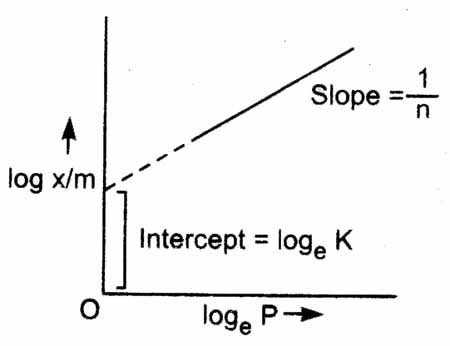Q

# I need help with For a linear plot of log (x/m) versus log p in a Freundlich adsorption isotherm, which of the following statements is correct ? (k and n are constants)

For a linear plot of log (x/m) versus log p in a Freundlich adsorption isotherm, which of the following statements is correct ?  (k and n are constants)

• Option 1)

Both k and 1/n appear in the slope term.

• Option 2)

1/n appears as the intercept.

• Option 3)

Only 1/n appears as the slope.

• Option 4)

log (1/n) appears as the intercept.

324 Views

As we learnt in

Logarithmic graph of Freundlich isotherm -- wherein

The slope of the straight line gives the value of 1/n. The intercept on y-axis gives the value of log K.

If a graph is plotted between log  and logP a staright line will be obtained.

Option 1)

Both k and 1/n appear in the slope term.

This option is incorrect.

Option 2)

1/n appears as the intercept.

This option is incorrect.

Option 3)

Only 1/n appears as the slope.

This option is correct.

Option 4)

log (1/n) appears as the intercept.

This option is incorrect.

Exams
Articles
Questions